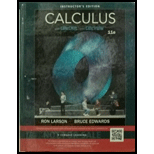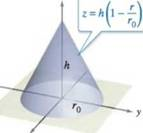Chapter 14.7, Problem 24E### Calculus (MindTap Course List)

11th Edition
Ron Larson + 1 other
ISBN: 9781337275347

#### Solutions

Chapter
Section### Calculus (MindTap Course List)

11th Edition
Ron Larson + 1 other
ISBN: 9781337275347
Textbook Problem

# Using Cylindrical Coordinates In Exercises 23-28, use cylindrical coordinates to find the indicated characteristic of the cone shown in the figure.Find the centroid of the cone.

To determine

To calculate: The centroid of the given cone, the cone has equation z=h(1rr0)

Explanation

Given:

The cylindrical coordinates of the cone indicated by z=h(1rr0) shown in the following figure:

Formula Used:

The mass of the solid is,

mass = volumedensity

The moments are found by,

Myz=Qxρ(x,y,z)dVMxz=Qyρ(x,y,z)dVMxy=Qzρ(x,y,z)dV

The volume is V=QdV

Also use the integration formula xndx=xn+1n+1

Calculation:

First, the volume of the cone using the cylindrical coordinates

V=Erdzdrdθ

Apply limits and use xndx=xn+1n+1

V=02π0r00h(1rr0)rdzdrdθ=02π0r0[rz]0h(1rr0)drdθ=02π0r0rh(1rr0)drdθ=hr002π0r0(rr0r2)drdθ

On further simplification,

V=hr002π[r22r0r33]0r0dθ=hr002πr036dθ=hr0[r036]02π=hr0[r0362π]

On calculation,

V=hr02π3

The mass is thus found to be for density ρ

M=ρV=ρhr02π3

From the diagram, it can be seen that the centroid is on the positive z-axis, thus x¯=0,y¯=0

### Still sussing out bartleby?

Check out a sample textbook solution.

See a sample solution

#### The Solution to Your Study Problems

Bartleby provides explanations to thousands of textbook problems written by our experts, many with advanced degrees!

Get Started

## Additional Math Solutions

#### Find more solutions based on key concepts